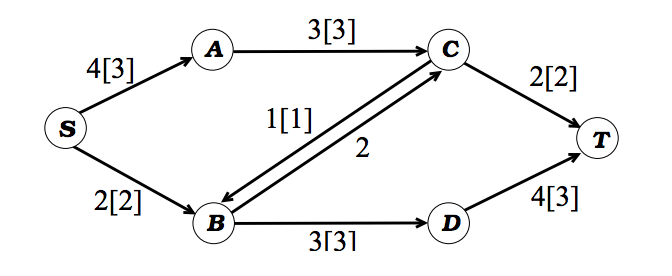Network flows is a class of problems dealing with directed graphs and the properties of functions defined on the graph.

# Flow

Flow represents any element which does not disappear while traveling through the edges of the directed graph. Flow can be current in the electric network, data packets in case of the computer network and vehicles in the transportation network.

# Maximum Flow

The goal of maximum flow problem is to maximize the flow from a given source vertex to a given sink vertex.

let’s define G = (V, E) be a directed graph, where is the set of vertices and is the set of edges in the given graph G.

The number of vertexes or the cardinality of is n. The number of edges or the cardinality of E is m.

N+(v)  is the set of endpoints of edges coming out of the vertex v.

N(v)  is the set of endpoints of edges coming into the vertex v.

Let a positive real number, u be defined as the maximum capacity of the edge of G.

Let’s define and together as the network.Figure 1. u is the number shown on the edges.S is the source. T is the sink. Arrows are the edges. A, B, C, D, S, T are the vertices.

## Raw Flow

A raw flow on a network is defined as function r, satisfying the following properties:

### Capacity Constraints

At every edge, the flow cannot be more than the capacity.

or in other words, for every edge is less than or equal to capacity, u.

### Conservation Constraints

At every vertex of source and sink, the flow into the vertex must equal the flow out from the vertex.Based on the above constraints, Can we find out the maximum flow from source, S to sink T in the network shown in figure 1?Figure 2. The number in brackets represents the optimum flow units to maximize the flow from S to T.

Based on the solution depicted in figure 2, the maximum flow is 5.

How do we know that 5 is indeed the right solution?

Let’s discuss what is the minimum total weight of edges that has to be removed in order to completely disconnect source, S from the sink, T?

I have listed some options among all :

1. Remove SA and SB = Total weight 6
2. Remove CT and DT = Total weight 6
3. Remove AC and SB = Total weight 5

If you go on listing all the options, you would verify that AC and SB is the minimum total weight that has to be removed in order to completely cut off S from T.

### s-t cut

An s-t cut is a set of edges whose removal disconnects t from s. Or, formally, a cut is a partition of the vertex set into two pieces A and B where s ∈ A and t ∈ B. (The edges of the cut are then all edges going from A to B).

### Max-flow min-cut theorem

In computer science and optimization theory, the max-flow min-cut theorem states that in a flow network, the maximum amount of flow passing from the source to the sink is equal to the total weight of the edges in the minimum cut, i.e. the smallest total weight of the edges which if removed would disconnect the source from the sink.

### Residual Capacity

Given a flow f in graph G, the residual capacity cf (u,v) is defined as cf (u,v) = c(u,v) − f(u,v), where recall that by skew-symmetry we have f(v,u) = −f(u,v).

In more simpler words, It’s the capacity remaining after the flow. Let’s take the example of SA.

SA has the capacity of 4. so, c = 4

SA has the flow of 3. so, f = 3

Residual capacity, the capacity remaining after the flow is = c- f =  4-3 = 1.

What is the residual capacity of AS? c of AS is 0. f is -3.

So, the residual capacity of AS is 0 – (-3) = 3

### Residual Graph

Given a flow f in graph G, the residual graph is the directed graph with all
edges of positive residual capacity, each one labeled by its residual capacity. Note: this may include back-edges of the original graph G.

Consider the graph in Figure 1 and suppose we push two units of flow on
the path s → b → c → t. We then end up with the following residual graph:Notice the back edge used. For example, the edge SB which was S to B in original graph but it’s residual graph has flow from B to S.

## Ford-Fulkerson algorithm

The Ford-Fulkerson algorithm is simply the following: while there exists an s → t path P of positive residual capacity, push the maximum possible flow along P.  These paths P are called augmenting paths because you use them to augment the existing flow.

This algorithm is an example of the greedy algorithm.